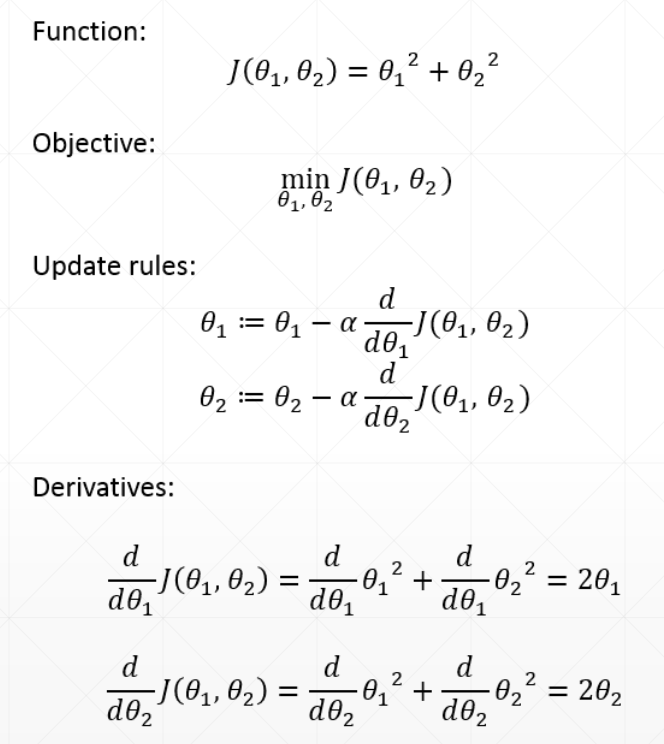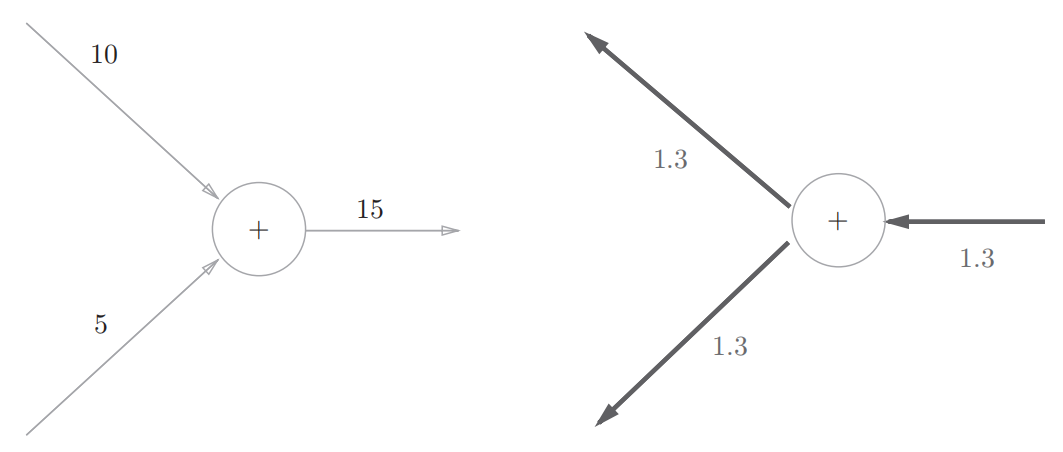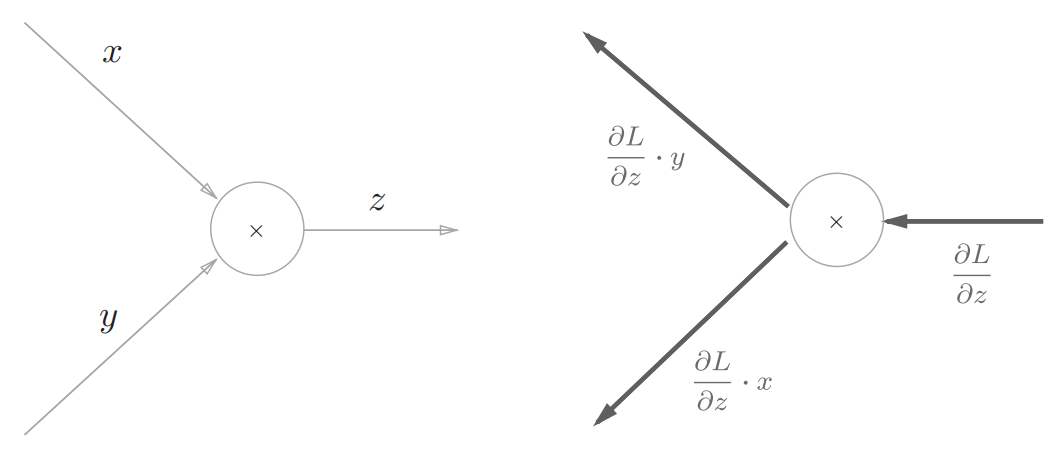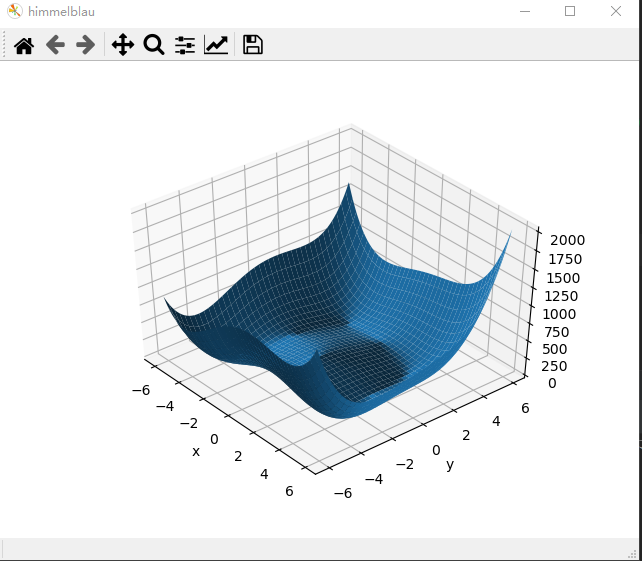• 如图： 优化问题 优化问题，有3种要考虑，初始化的值，学习率，优化方法（adam或者SGD） 以函数 z = ( x 2 + y − 11 ) 2 + ( x + y 2 − 7 ) 2 z=(x^2+y-11)^2+(x+y^2-7)^2 z=(x2+y−11)2+(x+y2−7)2为例子，利用...
6、梯度下降
了解3个定义 导数。偏导数，梯度 梯度下降搜索方法为：但这种搜索一般是局部最小或者最大值。 影响搜索过程的还有： 初试状态，学习率，动量
激活函数与Loss的梯度
import torch
from torch.nn import functional as F

mse = F.mse_loss(torch.ones(1), x * w)


做分类的话，最后还要用softmax函数， 好处是把原来大的值的比重放的更大
反向传播
一个能够高效计算权重参数的梯度的方法。计算反向传播需要用到高数里的链式法则。
加法节点的反向传播
因为加法节点的反向传播只乘以1，所以输入的值会原封不动地流向下一个节点。 如图：乘法节点的反向传播
乘法的反向传播会将上游的值乘以正向传播时的输入信号的“翻转值”后传递给下游。 如图：优化问题

z

=

(

x

2

+

y

−

11

)

2

+

(

x

+

y

2

−

7

)

2

z=(x^2+y-11)^2+(x+y^2-7)^2

为例子，利用梯度下降寻找最小值 代码：
import numpy as np
from mpl_toolkits.mplot3d import Axes3D
from matplotlib import pyplot as plt
import torch

def himmelblau(x):
return (x ** 2 + x - 11) ** 2 + (x + x ** 2 - 7) ** 2

x = np.arange(-6, 6, 0.1)
y = np.arange(-6, 6, 0.1)
print("x,y range:", x.shape, y.shape)
# np.meshgrid:生成网格点坐标矩阵
X, Y = np.meshgrid(x, y)
print("X,Y maps:", X.shape, Y.shape)
Z = himmelblau([X, Y])

fig = plt.figure('himmelblau')
# 绘制成3d图
ax = fig.gca(projection='3d')
ax.plot_surface(X, Y, Z)
ax.view_init(60, -30)
ax.set_xlabel('x')
ax.set_ylabel('y')
plt.show()

# 使用随机梯度下降的方法求解
# 初始化x的值为：[1., 0.], [-4, 0.], [4, 0.]
for step in range(20000):

pred = himmelblau(x)

pred.backward()
optimizer.step()

if step % 2000 == 0:
print ('step {}: x = {}, f(x) = {}'
.format(step, x.tolist(), pred.item()))

图片结果为：运行结果为：
x,y range: (120,) (120,)
X,Y maps: (120, 120) (120, 120)
step 0: x = [-3.999000072479248, -0.0009999999310821295], f(x) = 146.0
step 2000: x = [-3.526559829711914, -2.5002429485321045], f(x) = 19.4503231048584
step 4000: x = [-3.777446746826172, -3.2777843475341797], f(x) = 0.0012130826944485307
step 6000: x = [-3.7793045043945312, -3.283174753189087], f(x) = 5.636138666886836e-09
step 8000: x = [-3.779308319091797, -3.28318190574646], f(x) = 7.248672773130238e-10
step 10000: x = [-3.7793095111846924, -3.28318452835083], f(x) = 8.822098607197404e-11
step 12000: x = [-3.7793102264404297, -3.2831854820251465], f(x) = 8.185452315956354e-12
step 14000: x = [-3.7793102264404297, -3.2831859588623047], f(x) = 0.0
step 16000: x = [-3.7793102264404297, -3.2831859588623047], f(x) = 0.0
step 18000: x = [-3.7793102264404297, -3.2831859588623047], f(x) = 0.0

这里设置的初始值为（-4,0），如果初始值为（0,0）或者（4,0），则最小值虽然都是0，但x和y的值不一样，得出最后结果有4个不同的解对应的最小值0
8、过拟合与欠拟合
过拟合指的是只能拟合训练数据，但不能很好地拟合不包含在训练数据中的其他数据的状态。   机器学习的目标是提高泛化能力，即便是没有包含在训练数据里的未观测数据，也希望模型可以进行正确的识别。
过拟合
发生过拟合的原因，主要有以下两个。 1、模型拥有大量参数、表现力强。 2、训练数据少。
解决方法： 1、权值衰减是一直以来经常被使用的一种抑制过拟合的方法。该方法通过在学习的过程中对大的权重进行惩罚，来抑制过拟合。很多过拟合原本就是因为权重参数取值过大才发生的。 2、Dropout是一种在学习的过程中随机删除神经元的方法。训练时，随机选出隐藏层的神经元，然后将其删除。被删除的神经元不再进行信号的传递。训练时，每传递一次数据，就会随机选择要删除的神经元。然后，测试时，虽然会传递所有的神经元信号，但是对于各个神经元的输出，要乘上训练时的删除比例后再输出。
欠拟合
欠拟合就是模型没有很好地捕捉到数据特征，不能够很好地拟合数据。
解决方法： 1、添加其他特征项，有时是特征不够导致拟合的不好。 2、添加多项式特征，比如给线性模型添加二次或者多次项函数，使模型的泛化能力更强。 3、减少正则化参数，正则化是用来防止过拟合的，但是模型出现欠拟合后，则需要减少正则化参数。
9、交叉验证
在机器学习里，通常来说我们不能将全部用于数据训练模型，否则我们将没有数据集对该模型进行验证，从而评估我们的模型的预测效果。
解决方法：   把整个数据集分成两部分，一部分用于训练，一部分用于验证，这也就是我们经常提到的训练集（training set）和测试集（test set）。   一般采用k折交叉验证，假设k=10，步骤是： 1、将所有数据分成10； 2、不重复地每次取其中一份做测试集，用其他九份做训练集训练模型，之后计算该模型在测试集上的MSE； 3、将10次的MSE取平均，最后得到最终的MSE。
10、正则化
正则化有，L1正则化 和 L2正则化，或者叫作 L1范数 和 L2范数。   L1正则化和L2正则化可以看做是损失函数的惩罚项。所谓『惩罚』是指对损失函数中的某些参数做一些限制。对于线性回归模型，使用L1正则化的模型建叫做Lasso回归，使用L2正则化的模型叫做Ridge回归（岭回归）。   一般回归分析中w表示特征的系数，从上式可以看到正则化项是对系数做了处理（限制）。L1正则化和L2正则化的说明如下： L1：L1正则化是指权值向量w中各个元素的绝对值之和； L2：L2正则化是指权值向量w中各个元素的平方和然后再求平方根。   L1正则化和L2正则化的作用： 1、L1正则化可以产生稀疏权值矩阵，即产生一个稀疏模型，可以用于特征选择； 2、L2正则化可以防止模型过拟合（overfitting）；一定程度上，L1也可以防止过拟合。
展开全文神经网络
• 文章目录Pytorch 如何自动优化/调整 模型超参背景优化模型参数贝叶斯优化深度学习框架下的参数优化平台安装使用参考参考 背景 对于优化模型性能表现而言，主要可归纳为两种方式： 采用NAS的方式搜索，获得某个任务...
Pytorch 如何自动优化/调整 模型超参

文章目录
Pytorch 如何自动优化/调整 模型超参背景
优化模型参数贝叶斯优化
深度学习框架下的参数优化平台安装使用参考参考

背景
对于优化模型性能表现而言，主要可归纳为两种方式：
采用NAS的方式搜索，获得某个任务上最优的模型结构以及模型参数设置优化模型参数
优化模型参数
首先需要搞清楚，这里所指的优化模型参数是指在深度学习时代优化模型的超参。什么是超参了？超参是指，必须由人工设定的模型参数，比如学习率，比如mini-batch 的batch size，比如衰减率等等。参数优化特指的是这一类超参。

通常而言，在传统机器学习时代，针对一些结构较为简单的模型，比如随机数森林；其超参数量有限，并且超参的取值范围一般来说比较小，且并非连续数字；比如随机森林的depth参数只能是取整数；因而在这种情况下主要采用的是 网格搜索的方法即grid search 的方法进行合适超参的选取，即超参的优化。 很明显的是，采用grid search的方法不但浪费大量计算资源，并且效率无法保障。经过前人的验证，在传统机器学习时代形成了三种主要的调参方法：
grid searchrandom search（随机调参）bayesian optimize （贝叶斯调参/贝叶斯优化）
其中普遍来说，后两者的效果要更好；尤其是bayesian optimzie 性能更好，更加优异；在当时广泛用在诸如kaggle等比赛上(我曾经见过有kaggle的比赛团队使用贝叶斯调参将模型accuracy从76% 调到95%)。
贝叶斯优化
贝叶斯优化的主体思想是基于贝叶斯原理，即根据现有发生的事情（一组超参下模型表现情况）来推断如果采用另一组超参模型会是什么样的表现；简而言之就是基于少量的现有超参组合来估计整个超参组合空间，从中挑选出最为合适的超参组合；这个过程常常采用基于高斯回归来做估计；具体的原理可以参考链接。如果需要尝试下贝叶斯优化的效果，在使用传统算法时可以参考链接，这里有使用案例，可以配合sklearn 进行测试。我这里提供一段代码以供测试
# coding:utf-8

from sklearn.datasets import make_classification
from sklearn.ensemble import RandomForestClassifier
from sklearn.model_selection import cross_val_score
import numpy as np
from bayes_opt import BayesianOptimization

x,y = make_classification(n_samples=1000,n_features=10,n_classes=2)
rf = RandomForestClassifier()
#rf.fit(x,y)
print(np.mean(cross_val_score(rf,x,y,cv=20,scoring='roc_auc')))

def rf_cv(n_estimators,min_samples_split,max_features,max_depth):
'''这里面输入的参量全是超参'''
val = cross_val_score(
RandomForestClassifier(n_estimators=int(n_estimators),
min_samples_split=int(min_samples_split),
max_features=min(max_features,0.999),
max_depth=int(max_depth),
random_state=2),
x,
y,
scoring='roc_auc',cv=20
).mean()
return val

rf_bo = BayesianOptimization(rf_cv,
{'n_estimators': (10, 250),
'min_samples_split': (2, 25),
'max_features': (0.1, 0.999),
'max_depth': (5, 15)},
)

rf_bo.maximize()

深度学习框架下的参数优化
那么现在问题来了，在深度学习框架下，一个模型往往比之前复杂的多，且计算资源消耗得多；即便是使用贝叶斯方法，理论上需要运行的次数更少，但是也难以承担这样的开销。 针对这样的问题，普遍的做法是针对特定的框架开发专门的平台来进行优化。
平台安装
这里的平台安装部署非常简单，保持了pytorch 一贯的易于维护风格，直接使用pip 安装就可以了；安装过程可以参考 ax botorch 不过需要注意的是，上面两个平台需要基于torch 1.5 版本
使用参考
使用AX 和 Botorch 优化我们自定义的模型是第一步，这里给出一段代码以供参考
class VAE(nn.Module):
def __init__(self):
super().__init__()
self.fc1 = nn.Linear(784, 400)
self.fc21 = nn.Linear(400, 20)
self.fc22 = nn.Linear(400, 20)
self.fc3 = nn.Linear(20, 400)
self.fc4 = nn.Linear(400, 784)

def encode(self, x):
h1 = F.relu(self.fc1(x))
return self.fc21(h1), self.fc22(h1)

def reparameterize(self, mu, logvar):
std = torch.exp(0.5*logvar)
eps = torch.randn_like(std)
return mu + eps*std

def decode(self, z):
h3 = F.relu(self.fc3(z))

def forward(self, x):
mu, logvar = self.encode(x.view(-1, 784))
z = self.reparameterize(mu, logvar)
return self.decode(z), mu, logvar

vae_model = VAE().to(device)

从上面可以看得出，这就是一个普通的pytorch 模型结构定义的方式,接下来就是构建贝叶斯优化要优化的目标函数，这当然是一个黑盒过程。这个函数其实也就是用于描述模型表现的一个函数
def score(y):
"""Returns a 'score' for each digit from 0 to 9. It is modeled as a squared exponential
centered at the digit '3'.
"""

# Given the scoring function, we can now write our overall objective, which as discussed above, starts with an image and outputs a score. Let's say the objective computes the expected score given the probabilities from the classifier.

# In:

def score_image_recognition(x):
"""The input x is an image and an expected score based on the CNN classifier and
the scoring function is returned.
"""
probs = torch.exp(cnn_model(x))  # b x 10
scores = score(torch.arange(10, device=device, dtype=dtype)).expand(probs.shape)
return (probs * scores).sum(dim=1)

# Finally, we define a helper function decode that takes as input the parameters mu and logvar of the variational distribution and performs reparameterization and the decoding. We use batched Bayesian optimization to search over the parameters mu and logvar

# In:

def decode(train_x):
decoded = vae_model.decode(train_x)
return decoded.view(train_x.shape, 1, 28, 28)

然后就是使用Botorch 和Ax进行模型贝叶斯优化的过程，这个过程分为4个主体步骤，下面代码中一一介绍
# 1.  定义每一次的模型初始化
from gpytorch.mlls.exact_marginal_log_likelihood import ExactMarginalLogLikelihood

bounds = torch.tensor([[-6.0] * 20, [6.0] * 20], device=device, dtype=dtype)

def initialize_model(n=5):
# generate training data
train_x = (bounds - bounds) * torch.rand(n, 20, device=device, dtype=dtype) + bounds
train_obj = score_image_recognition(decode(train_x))
best_observed_value = train_obj.max().item()

# define models for objective and constraint
model = model.to(train_x)

mll = ExactMarginalLogLikelihood(model.likelihood, model)
mll = mll.to(train_x)

return train_x, train_obj, mll, model, best_observed_value

from botorch.optim import joint_optimize

BATCH_SIZE = 3

# 2. 定义要优化的参量
def optimize_acqf_and_get_observation(acq_func):
"""Optimizes the acquisition function, and returns a new candidate and a noisy observation"""

# optimize 这里定义的调的超参是batch size
candidates = joint_optimize(
acq_function=acq_func,
bounds=bounds,
q=BATCH_SIZE,
num_restarts=10,
raw_samples=200,
)

# observe new values
new_x = candidates.detach()
new_obj = score_image_recognition(decode(new_x))
return new_x, new_obj

# 3. 定义优化过程
from botorch import fit_gpytorch_model
from botorch.acquisition.monte_carlo import qExpectedImprovement
from botorch.sampling.samplers import SobolQMCNormalSampler

seed=1
torch.manual_seed(seed)

N_BATCH = 50
MC_SAMPLES = 2000
best_observed = []

# call helper function to initialize model
train_x, train_obj, mll, model, best_value = initialize_model(n=5)
best_observed.append(best_value)

# 4. 开始进行 优化
import warnings
warnings.filterwarnings("ignore")

print(f"\nRunning BO ", end='')
from matplotlib import pyplot as plt

# run N_BATCH rounds of BayesOpt after the initial random batch
for iteration in range(N_BATCH):

# fit the model
fit_gpytorch_model(mll)

# define the qNEI acquisition module using a QMC sampler
qmc_sampler = SobolQMCNormalSampler(num_samples=MC_SAMPLES, seed=seed)
qEI = qExpectedImprovement(model=model, sampler=qmc_sampler, best_f=best_value)

# optimize and get new observation
new_x, new_obj = optimize_acqf_and_get_observation(qEI)

# update training points
train_x = torch.cat((train_x, new_x))
train_obj = torch.cat((train_obj, new_obj))

# update progress
best_value = score_image_recognition(decode(train_x)).max().item()
best_observed.append(best_value)

# reinitialize the model so it is ready for fitting on next iteration
model.set_train_data(train_x, train_obj, strict=False)

print(".", end='')

基本来说，采用Botorch Ax进行优化 就是上面的4个步骤，主要是要把优化的参数在candidates里面列好。 上述的步骤是针对一般性的优化函数，比距高斯回归优化函数的步骤，Botorch 还支持自定义的RBF优化函数；关于这一块的内容可以具体参考Botorch的相关文档。目前有一些研究结果表明一些特定的RBF函数有较好的优化效果。
参考
https://www.cnblogs.com/marsggbo/p/9866764.htmlGoogle Vizier: A Service for Black-Box OptimizationEfficient Hyperparameter Optimization of Deep Learning Algorithms Using Deterministic RBF Surrogatesbotorch:programmable bayesian opt in pytorch
展开全文深度学习 神经网络
• 为了将这两个领域的精华结合在一起，我们开发了Auto-PyTorch ，它联合稳健地优化网络架构和训练超参数，以实现全自动深度学习 (AutoDL)。 Auto-PyTorch 主要用于支持表格数据（分类、回归），但也可以应用于图像...Python
• Talos通过完全自动化的超参数调整和模型评估，从根本上改变了普通的Keras工作流程。 Talos完全公开了Keras功能，没有新的语法或模板可学习。 塔罗斯 TL; DR Talos可以从根本上改变普通的Keras工作流程，而不会占用...
torch.nn是专门为神经网络设计的模块化接口。模块化。
# 引入torch.nn并指定别名,一般别名命名为nn
import torch.nn as nn

torch.nn只支持mini-batches，不支持一次只输入一个样本，即一次必须是一个batch。也就是说，就算我们输入一个样本，也要增加一维变成批。
可学习参数： 网络的可学习参数通过net.parameters()返回，返回值是一个可迭代对象，或者说列表，列表里面放的是参数对象Parameter，参数对象里面主要就包含了一个tensor。 net.named_parameters可同时返回可学习的参数及名称。这个就相当于返回的dict，可以方便的知道名字。
Net(
(fc1): Linear(in_features=2, out_features=4, bias=True)
)
('fc1.weight', Parameter containing:
tensor([[-0.5767,  0.1687],
[ 0.0383, -0.0288],
[ 0.1512,  0.3680],
('fc1.bias', Parameter containing:


这是一个只有一个2*4 linear层的，可见线性层的参数分为了两部分，本身的参数和偏置参数， 偏置参数和输出层的数量相同，就是每个单元对应到一个参数。a = wx+b
比较奇怪的是，并没有明确的指明模型每层是什么样的，pytorch却知道了可学习参数是什么。它应该是通过类包含的属性来获取的，就是通过self.xxx = xxx 中给类指定的属性来知道了参数。 这里面有几个点要了解的 1、属性中创建的层的顺序必须和前向传播的顺序一样吗？这应该是因为反向时会按照层被调用的顺序计算梯度，所以创建顺序对反向传播梯度值计算没有影响。然后，根据梯度更新参数，是否受到参数顺序影响，这个不知道。不过按照pytorch这种架构，应该就是不会影响了，也就是说更新参数只会利用到改层参数和他的梯度，不会利用前后层的参数和梯度。由上，更新梯度时，可以和调用顺序不一样。 2、通过属性创建的层，和前向传播中用到的层，必须保持一致吗，也就是说创建的层都必须在前向传播中使用吗？或者没创建的层能在前向传播中使用吗？根据梯度更新的方式，如果没有被使用，那么改层的梯度不存在或者为0，不会对其参数产生影响。所以，应该是可以不是用的。然后没创建的层在前向传播中使用那是语法错误，不存在这种情况。所以这两个地方不比保持一致。
优化器
learning_rate = 0.01
for f in net.parameters():

展开全文• pytorch优化器： 管理并更新模型中可学习参数的值，使得模型输出更接近 真实标签 导数：函数在指定坐标轴上的变化率 方向导数：指定方向上的变化率 梯度：一个向量，方向为方向导数取得最大值的方向 基本属性 • ...


优化器
一、优化器属性与方法二、pytorch中的优化器

一、优化器属性与方法
pytorch的优化器： 管理并更新模型中可学习参数的值，使得模型输出更接近 真实标签
导数：函数在指定坐标轴上的变化率 方向导数：指定方向上的变化率 梯度：一个向量，方向为方向导数取得最大值的方向基本属性 • defaults：优化器超参数 • state：参数的缓存，如momentum的缓存 • params_groups：管理的参数组，list中有个字典 • _step_count：记录更新次数，学习率调整中使用
基本方法 • zero_grad()：清空所管理参数的梯度 pytorch特性：张量梯度不自动清零• zero_grad()：清空所管理参数的梯度 • step()：执行一步更新
• zero_grad()：清空所管理参数的梯度 • step()：执行一步更新 • add_param_group()：添加参数组• zero_grad()：清空所管理参数的梯度 • step()：执行一步更新 • add_param_group()：添加参数组
常用在优化器参数的保存与加载 • state_dict()：获取优化器当前状态信息字典 • load_state_dict() ：加载状态信息字典学习率（learning rate）: 控制更新的步伐
Momentum（动量，冲量）： 结合当前梯度与上一次更新信息，用于当前更新
让更新保持一个惯性

指数加权平均:

v

t

=

β

∗

v

t

−

1

+

(

1

−

β

)

∗

θ

t

v

100

=

β

∗

v

99

+

(

1

−

β

)

∗

θ

100

=

(

1

−

β

)

∗

θ

100

+

β

∗

(

β

∗

v

98

+

(

1

−

β

)

∗

θ

99

)

=

(

1

−

β

)

∗

θ

100

+

(

1

−

β

)

∗

β

∗

θ

99

+

(

β

2

∗

v

98

)

=

(

1

−

β

)

∗

θ

100

+

(

1

−

β

)

∗

β

∗

θ

99

+

(

1

−

β

)

∗

β

2

∗

θ

98

+

(

β

3

∗

v

97

)

=

(

1

−

β

)

∗

β

0

∗

θ

100

+

(

1

−

β

)

∗

β

1

∗

θ

99

+

(

1

−

β

)

∗

β

2

∗

θ

98

+

(

β

3

∗

v

97

)

得到通式：

=

∑

i

N

(

1

−

β

)

∗

β

i

∗

θ

N

−

i

\begin{array}{l} \text { 指数加权平均: } \quad \mathrm{v}_{t}=\beta * v_{t-1}+(1-\beta) * \theta_{t} \\ \mathrm{v}_{100}=\beta * v_{99}+(1-\beta) * \theta_{100} \\ =(1-\beta) * \theta_{100}+\beta *\left(\beta * v_{98}+(1-\beta) * \theta_{99}\right) \\ =(1-\beta) * \theta_{100}+(1-\beta) * \beta * \theta_{99}+\left(\beta^{2} * v_{98}\right) \\ =(1-\beta) * \theta_{100}+(1-\beta) * \beta * \theta_{99}+(1-\beta) * \beta^{2} * \theta_{98}+\left(\beta^{3} * v_{97}\right) \\ =(1-\beta) * \beta^{0}* \theta_{100}+(1-\beta) * \beta^{1} * \theta_{99}+(1-\beta) * \beta^{2} * \theta_{98}+\left(\beta^{3} * v_{97}\right) \\ \text{得到通式：}\\ =\sum_{i}^{N}(1-\beta) * \beta^{i} * \theta_{N-i} \end{array}

beta越小记忆周期越短原始梯度下降公式：

w

i

+

1

=

w

i

−

l

r

∗

g

(

w

i

)

w_{i+1}=w_{i}-l r * g\left(w_{i}\right)

pytorch中更新公式：

v

i

=

m

∗

v

i

−

1

+

g

(

w

i

)

w

i

+

1

=

w

i

−

l

r

∗

v

i

\begin{array}{c} v_{i}=m * v_{i-1}+g\left(w_{i}\right) \\ w_{i+1}=w_{i}-l r * v_{i} \end{array}

𝒘𝒊+𝟏：第i+1次更新的参数   lr：学习率   𝒗𝒊 ：更新量   m：momentum系数   𝒈(𝒘𝒊)： 𝒘𝒊的梯度 eg:

v

100

=

m

∗

v

99

+

g

(

w

100

)

=

g

(

w

100

)

+

m

∗

(

m

∗

v

98

+

g

(

w

99

)

)

=

g

(

w

100

)

+

m

∗

g

(

w

99

+

m

2

∗

v

98

=

g

(

w

100

)

+

m

∗

g

(

w

99

)

+

m

2

∗

g

(

w

98

)

+

m

3

∗

v

97

\begin{aligned} v_{100} &=m * v_{99}+g\left(w_{100}\right) \\ &=g\left(w_{100}\right)+m *\left(m * v_{98}+g\left(w_{99}\right)\right) \\ &=g\left(w_{100}\right)+m * g\left(w_{99}+m^{2} * v_{98}\right.\\ &=g\left(w_{100}\right)+m * g\left(w_{99}\right)+m^{2} * g\left(w_{98}\right)+m^{3} * v_{97} \end{aligned}

二、pytorch中的优化器
optim.SGD pytorch中最常用也是最实用的优化器 主要参数： • params：管理的参数组 • lr：初始学习率 • momentum：动量系数，贝塔 • weight_decay：L2正则化系数 • nesterov：是否采用NAG
optim.SGD(params, lr=<object object>, momentum=0,
dampening=0, weight_decay=0, nesterov=False)

optim.Adagrad：自适应学习率梯度下降法optim.RMSprop： Adagrad的改进optim.Adadelta： Adagrad的改进optim.Adam：RMSprop结合Momentum， 《Adam: A Method for Stochastic Optimization》optim.Adamax：Adam增加学习率上限optim.SparseAdam：稀疏版的Adamoptim.ASGD：随机平均梯度下降optim.Rprop：弹性反向传播optim.LBFGS：BFGS的改进
展开全文深度学习
• 二、pytorch优化器——Optimizer 各种优化器都在optim包中，eg optim.SGD() 基本属性：defaults：优化参数,（net.parameters(),学习率,momentum=0.9(动量，结合当前梯度与上一次更新信息，用于当前更新
• 一、优化器：optimizer 管理并更新模型中可学习参数的值，使得模型输出更接近真实标签 ； 导数：函数在指定坐标轴上的变化率；...defaults：优化器超参数 state：参数的缓存，如momentum的缓存 ...optimizer python 深度学习
•机器学习 神经网络 深度学习
• Pytorch 一共有11个优化器，其中比较常用的主要有4种：SGD、Momentum、RMSProp、Adam。 SGD 实现随机梯度下降。 ...params (iterable) –待优化参数的iterable或者是定义了参数组的dict lr (float)深度学习 神经网络
• 训练数据的终极目的是得到一个训练好的模型，即这个模型里面的参数是最优的，也就是这些参数使得损失函数达到了最小，或者说模型收敛了（参数不再改变了） 二、 一阶优化算法 什么是梯度： 梯度就是导数，梯度是...
• 这就是Pytorch自动微分机制。 一，利用backward方法求导数 backward 方法通常在一个标量张量上调用，该方法求得的梯度将存在对应自变量张量的grad属性下。 如果调用的张量非标量，则要传入一个和它同形状 的...数据挖掘 机器学习 深度学习
• 文章目录PyTorch自动混合精度训练(AMP)手册AutocastingGradient ScalingNotesAutocast Op Look-up-tableReference PyTorch自动混合精度训练(AMP)手册 自动混合精度 —— Automatic Mixed Precision, AMP 混合精度...
• 1.torch.nn.parameter概要 ...parameters是张量的子类，当与模块s一起使用时，它们有一个非常特殊的属性——当它们被重新分配为模块属性时，它们会自动地添加到它的参数列表中，并且会出现在Parame...
• 点击上方“机器学习与生成对抗网络”，关注星标获取有趣、好玩的前沿干货！选自efficientdl.com，作者：LORENZ KUHN机器之心编译本文介绍在使用 PyTorch 训练深度...神经网络 人工智能 深度学习 tensorflow 计算机视觉
• 【导读】AutoML-Freiburg在Github上发布了PyTorch机器学习自动化的资源，可以自动框架搜索，超参数优化。作者强调这是一个 very early pre-alpha版本，目前只支持特征化数据。不过这不妨碍我们搓手试试~   安装...机器学习
• 可能的条件下，尽量使用in_place实现2.Pytorch的 ‘checkpoint’3.删除loss等不再被需要的值，释放对应的空间4. 混合精度训练 参考资料： 知乎讨论； pytorch论坛； 官方文档； 1.可能的条件下，尽量使用in_place...深度学习 人工智能
• pytorch自动编码Welcome to Part 2 of our series, where we shall start coding Proximal Policy Optimization (PPO) from scratch with PyTorch. If you haven’t read Part 1, please do so first.欢迎来到本系列...
• 自动编码器作为一种数据压缩的方法，其原理是：输入数据经过编码器变成一个编码（code），然后将这个编码作为解码器的输入，观察解码器的输出是否能还原原始数据，因此将解码器的输出和原始数据的误差作为最优化的...图片压缩
• 本文针对python的pytorch库中的自动求梯度进行了详细的解释 Tensor pytorch里面的tensor可以用来存储向量或者标量。 torch.tensor(1) # 标量 torch.tensor() # 1*1 的向量 tensor还可以指定数据类型，以及...深度学习 神经网络 机器学习 人工智能 python
• 参考资料 https://pytorch.org/ https://github.com/pytorch https://github.com/pytorch/pytorch ...PyTorch中文文档 https://github.com/awfssv/pytorch-cn 一、Day 1 1、学习内容 pyto...
• pytorch进阶学习零 [星期八的博客](https://theeighthday.github.io/2019/09/18/learn-pytorch/)一 利用Variable自动求导1.1 Variable1.1.1 定义1.1.2 特性1.2 自动求导过程1.2.1 输出为标量1.2.2 输出为向量1.2.2.1...
• 一组脚本，可通过RESTful API帮助新手为PyTorch进行数据扩充和超参数优化。 通过RESTful API，可以灵活地添加所需的GUI或Web界面，以实时可视化培训。 该库通过Redis Queue支持异步作业，并通过SocketIO支持实时...
• pytorch优化器：管理并更新模型中可学习参数的值，是的模型输出更接近真实标签。 导数：函数在指定坐标轴上的变化率 方向导数：指定方向上的变化率 梯度：一个响亮，方向为方向导数取得最大值的方向 pytorch中的...
• 自动求导与逻辑回归自动求导逻辑回归 自动求导 retain_graph设为True,可以进行两次反向传播 逻辑回归 import torch import torch.nn as nn import matplotlib.pyplot as plt import numpy as np torch.manual_...
• # scaler.step() 首先会 unscale 优化器指定参数的梯度。 # 如果这些梯度中没有 inf 或者 NaN，那么调用 optimizer.step() # 否则跳过 optimizer.step() scaler.step(optimizer) # Updates the scale ...python
• pytorch贝叶斯网络Hyperparameters are the parameters in models that determine model architecture, learning speed and scope, and regularization.超参数是确定模型架构，学习速度和范围以及正则化的模型中的...java 人工智能 python 机器学习 linux...